# Search

About 58 Search Results Matching Types of Worksheet, Worksheet Section, Generator, Generator Section, Grades matching 5th Grade, Similar to Back to School Cut and Paste Puzzle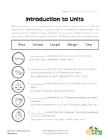## Introduction to Units of Measurement

Fill in the blanks with the correct unit type to m...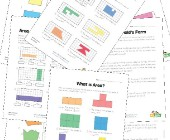## Area and Perimeter Worksheets

Help kids learn to calculate the area and perimete...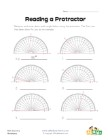## Reading a Protractor Worksheet 1

Read each protractor and write down the angles the...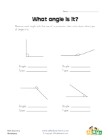## What Angle Is It?

Measure each angle and decide what type of angle i...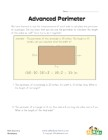Learn to use the perimeter to calculate the length...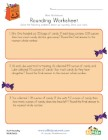## Halloween Rounding Worksheet

Solve the Halloween themed problems by rounding.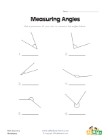## Measuring Angles Worksheet 2

Use a protractor to measure each of the angles.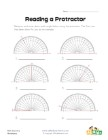## Reading a Protractor Worksheet 2

Read each protractor and write down the angles the...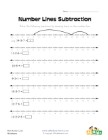## Number Lines Subtraction Worksheet 1

Use the number lines to solve the subtraction prob...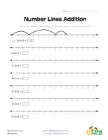## Number Lines Addition Worksheet 1

Use the number lines to solve the addition problem...